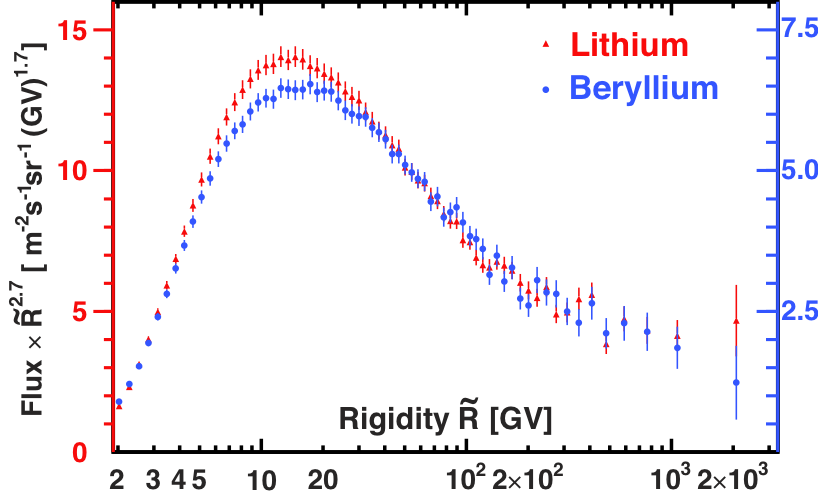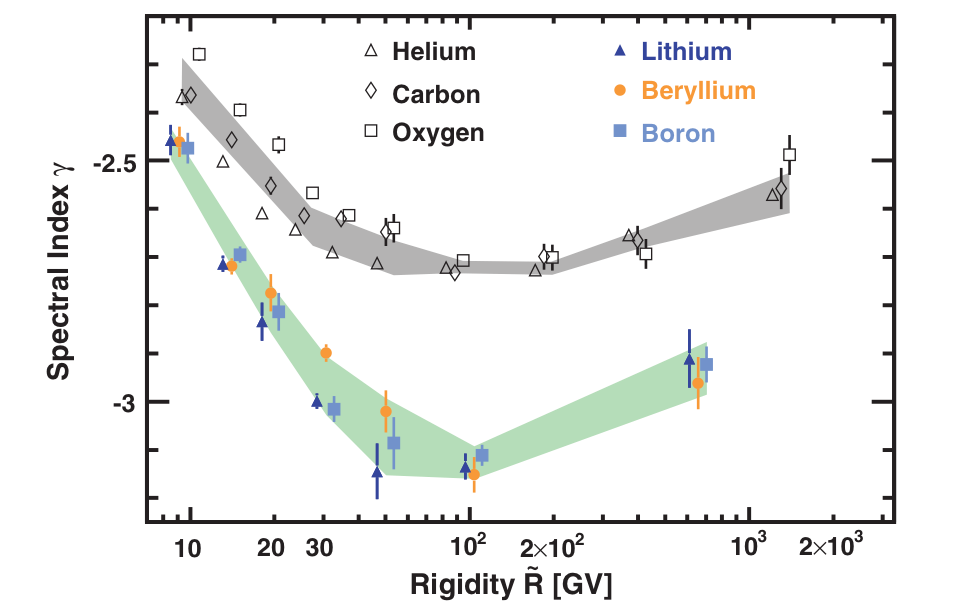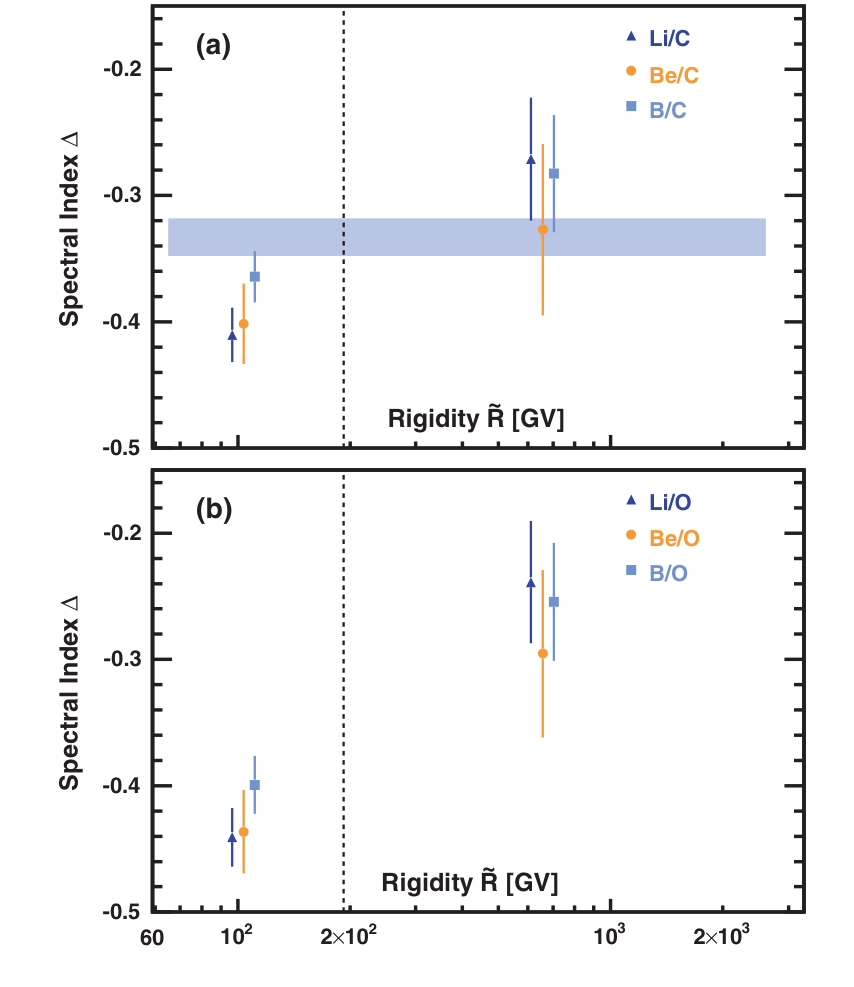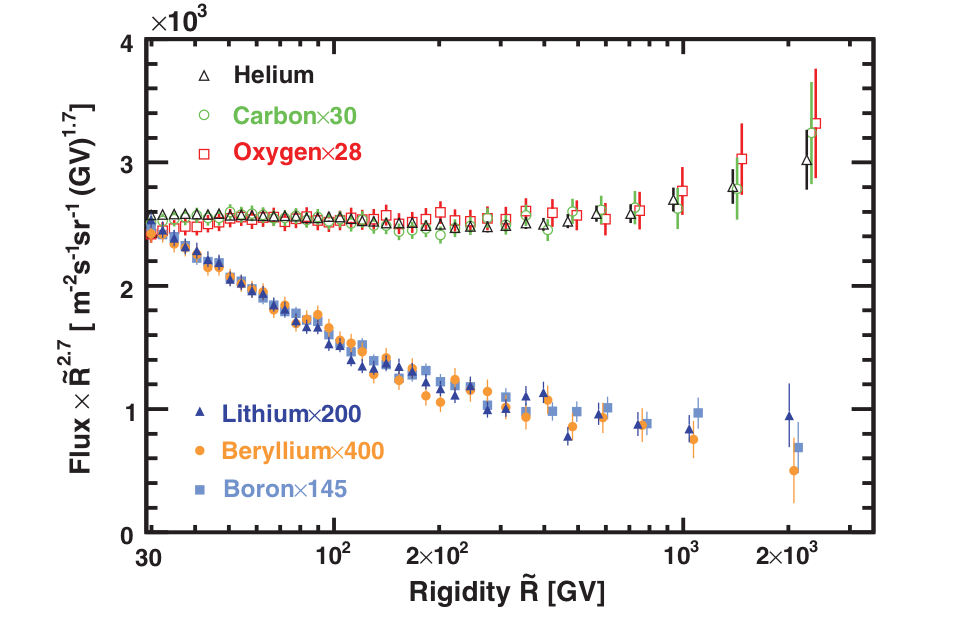# Observation of New Properties of Secondary Cosmic Rays Lithium, Beryllium and Boron

Lithium, beryllium, and boron nuclei in cosmic rays are thought to be produced by the collisions of nuclei with the interstellar medium. They are called secondary cosmic rays. Precise knowledge of their spectra in the GV-TV rigidity region provides important information on the propagation of cosmic rays.

AMS published the precision measurement of the lithium, beryllium, and boron fluxes in cosmic rays in the rigidity range from 1.9 GV to 3.3 TV. This measurement is based on 1.9 million lithium, 0.9 million beryllium, and 2.6 million boron nuclei collected by AMS during the first 5 years of operation aboard the International Space Station (ISS). The total error on each of the fluxes is 3%–4% at 100 GV.  Figure 1 shows the fluxes of lithium, beryllium and boron measured by AMS.Figure 1. The AMS (a) Li (red, right axis) and B (blue, left axis); and (b) Be (orange, right axis) and B (blue, left axis) fluxes multiplied by $\tilde{R}^{2.7}$ with their total errors as a function of rigidity.

As seen, the Li and B fluxes have an identical rigidity dependence above ∼7 GV and all three secondary fluxes have an identical rigidity dependence above ∼30 GV with the Li/Be flux ratio of $2.0 \pm 0.1$, as shown in Figure 2. Note that the different rigidity dependence of the Be flux and Li fluxes below 30 GV is due to the significant presence of the radioactive $\mathrm{^{10}Be}$ isotope, which has a half-life of 1.4 million years.Figure 2. The AMS Li (red, left axis) and Be (blue, right axis) fluxes multiplied by $\tilde{R}^{2.7}$ with their total errors as a function of rigidity. As seen, above 30 GV, the Li and Be fluxes have identical rigidity dependence and their ratio is $2.0 \pm 0.1$.

Precise measurements of primary cosmic rays helium, carbon, and oxygen, by AMS, have shown a hardening of all their spectra above 200 GV. In addition, above 60 GV, the spectra of He, C, and O were found to have an identical rigidity dependence [M. Aguilar et al., Phys. Rev. Lett. 119, 251101 (2017)].

The detailed knowledge of lithium, beryllium, and boron flux rigidity dependence is important to study the origin of the hardening in cosmic ray fluxes.

There are many theoretical models describing the behavior of cosmic rays. For example, if the hardening in cosmic rays is related to the injected spectra at their source, then similar hardening is expected both for secondary and primary cosmic rays [S. Thoudam and J. R. Hörandel, Mon. Not. R. Astron. Soc. 435, 2532 (2013)]. However, if the hardening is related to propagation properties in the Galaxy then a stronger hardening is expected for the secondary with respect to the primary cosmic rays [A. E. Vladimirov, G. Jóhannesson, I. V. Moskalenko, and T. A. Porter, Astrophys. J. 752, 68 (2012)]. The theoretical models have their limitations, as none of them predicted the AMS observed spectral behavior of the primary cosmic rays He, C, and O nor the secondary cosmic rays Li, Be, and B.

To examine the rigidity dependence of the secondary fluxes, detailed variations of the flux spectral indices with rigidity were obtained in a model-independent way. The lithium, beryllium and boron flux $\Phi$ spectral indices γ were calculated from $\gamma = d[\log(\Phi)/d[\log(R)]$ over rigidity intervals bounded by 7.09, 12.0, 16.6, 22.8, 41.9, 60.3, 192, and 3300 GV. The results are presented in Figure 3 together with the spectral indices of helium, carbon, and oxygen [M. Aguilar et al., Phys. Rev. Lett. 119, 251101 (2017)]. As seen, the magnitude and the rigidity dependence of the lithium, beryllium, and boron spectral indices are nearly identical, but distinctly different from the rigidity dependence of helium, carbon, and oxygen. In addition, above ∼200 GV, Li, Be, and B all harden more than He, C, and O.

This observed behavior is completely unexpected.Figure 3. The dependence of the Li, Be, and B spectral indices on rigidity together with the rigidity dependence of the He, C, and O spectral indices. For clarity, the Li, B, He, and O data points are displaced horizontally. The shaded regions are to guide the eye.

To examine the difference between the rigidity dependence of primary and secondary cosmic rays in detail, the ratios of the lithium, beryllium, and boron fluxes to the carbon and oxygen fluxes were computed using the corresponding flux values. The detailed variations with rigidity of the spectral indices $\Delta$ of the secondary to primary flux ratios $\Phi_{S}/\Phi_{P}$ were obtained in a model independent way using $\Delta = d[\log(\Phi_{S}/\Phi_{P})/d[\log(R)]$ over rigidity intervals [60.3 – 192] and [192 – 3300] GV and shown in Figure 4.  Above ∼200 GV these spectral indices exhibit hardening of $0.13 \pm 0.03$ on average. This shows that at high rigidities the secondary cosmic rays harden more than the primary cosmic rays. This additional hardening of secondary cosmic rays is consistent with expectations when the hardening is due to the propagation in the Galaxy. This is a new observation.Figure 4. The AMS secondary to primary flux ratio spectral indices Δ as functions of rigidity for (a) Li/C, Be/C, and B/C. The horizontal band indicates the fit to the B/C ratio from our previous publication [Precision Measurement of the Boron to Carbon Flux Ratio]. (b) The results for Li/O, Be/O, and B/O. For (a) and (b) the vertical dashed line shows the interval boundary. On average, the spectral indices of Li/C, Be/C, B/C, Li/O, Be/O, and B/O above 200 GV exhibit a hardening of $0.13\pm0.03$.

Figure 5 shows a comparison of the secondary cosmic ray fluxes Li, Be and B with the AMS primary cosmic ray fluxes He, C and O.  As seen, the three secondary fluxes have an identical rigidity dependence above 30 GV, as do the three primary fluxes above 60 GV. The rigidity dependences of primary cosmic ray fluxes and of secondary cosmic ray fluxes are distinctly different.Figure 5. Comparison of the secondary cosmic ray fluxes with the AMS primary cosmic ray fluxes multiplied by $\tilde{R}^{2.7}$ with their total error as a function of rigidity above 30 GV. For display purposes only, the C, O, Li, Be, and B fluxes were rescaled as indicated. For clarity, the He, O, Li, and B data points above 400 GV are displaced horizontally.

In conclusion, the precise, high statistics measurements of the lithium, beryllium, and boron fluxes from 1.9 GV to 3.3 TV show that the Li and B fluxes have identical rigidity dependence above 7 GV and all three fluxes have identical rigidity dependence above 30 GV with the Li/Be flux ratio of $2.0 \pm 0.1$. The three fluxes deviate from a single power law above 200 GV in an identical way. As seen in Figure 5, this behavior of secondary cosmic rays has also been observed in primary cosmic rays He, C, and O but the rigidity dependences of primary cosmic rays and of secondary cosmic rays are distinctly different. In particular, above 200 GV, the spectral indices of secondary cosmic rays harden by an average of $0.13 \pm 0.03$ more than the primaries. These are new properties of high energy cosmic rays.

Publication
Editors' Suggestion
Featured in Physics